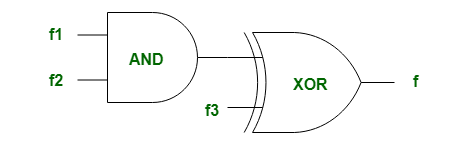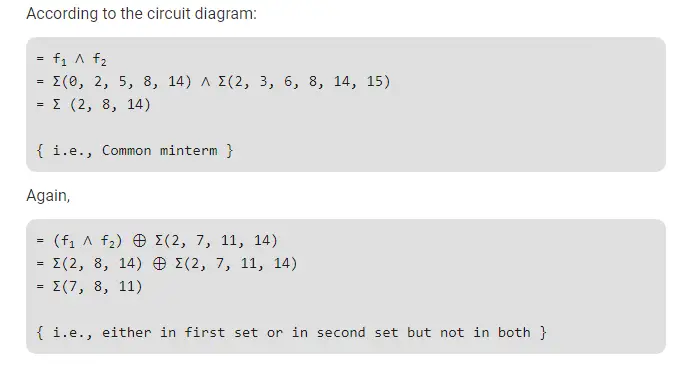# Gate CS-2019 Question Paper With Solutions

Q. 39 Consider three 4-variable functions f1, f2 and f3, which are expressed in sum-of-minterms

```f1 = Σ(0, 2, 5, 8, 14)
f2 = Σ(2, 3, 6, 8, 14, 15)
f3 = Σ(2, 7, 11, 14)```

For the following circuit with one AND gate and one XOR gate, the output function f can be expressed as:(A) Σ(7, 8, 11)

(B) Σ(2, 14)

(C) Σ(0, 2, 3, 5, 6, 7, 8, 11, 14)

(D) Σ(2, 7, 8, 11, 14)